This is an example of exploratory latent class analysis (LCA) with continuous indicators, otherwise known as latent profile analysis (LPA) or finite Gaussian mixture modeling, using tidySEM. See Van Lissa, C. J., Garnier-Villarreal, M., & Anadria, D. (2023). Recommended Practices in Latent Class Analysis using the Open-Source R-Package tidySEM. Structural Equation Modeling. https://doi.org/10.1080/10705511.2023.2250920. The present example uses data collected by Alkema as part of a study on ocean microplastics. The purpose of this study was to provide a more nuanced model for the distribution of different sizes of ocean microplastics than the commonly used normal distribution. To this end, a mixture of normals was used. Since there is no theoretical reason to expect a certain number of classes, this is an exploratory LCA. To view its documentation, run the command ?tidySEM::alkema_microplastics in the R console. The original analyses are available at https://github.com/cjvanlissa/lise_microplastics; in this vignette, we take a different approach to the analysis to showcase other possibilities.

To load the data, simply attach the tidySEM package. For convenience, we assign the variables used for analysis to an object called df. As explained in the paper, the classes are quite different for lines, films, and fragments. For this reason, we here only use data from fragments. The indicators are fragments’ length and width in millimeters. The sample size was not planned.

# Load required packages
library(tidySEM)
library(ggplot2)
df_analyze <- alkema_microplastics[alkema_microplastics$category == "Fragment", ] df <- df_analyze[, c("length", "width")] ## Descriptive statistics As per the best practices, the first step in LCA is examining the observed data. We use tidySEM::descriptives() to describe the data numerically. Because all items are continuous, we remove columns for categorical data to de-clutter the table: desc <- tidySEM::descriptives(df) desc <- desc[, c("name", "type", "n", "unique", "mean", "median", "sd", "min", "max", "skew_2se", "kurt_2se")] knitr::kable(desc, caption = "Descriptive statistics") Descriptive statistics name type n unique mean median sd min max skew_2se kurt_2se length numeric 5605 2086 2.9 2.4 1.9 1.0 69.2 137 2116 width numeric 5605 2079 2.0 1.6 1.1 0.2 6.8 22 37 The data are correctly coded as numeric and the distributional characteristics match the intended measurement level. The variable scales are comparable (both in millimeters and no large discrepancies between variances). There are no missing values; if any variables had missing values, we would report an MCAR test with mice::mcar(), and explain that missing data are accounted for using FIML. Additionally, we can plot the data. The ggplot2 function geom_density() is useful to visualize continuous data: df_plot <- df names(df_plot) <- paste0("Value.", names(df_plot)) df_plot <- reshape(df_plot, varying = names(df_plot), direction = "long", timevar = "Variable") ggplot(df_plot, aes(x = Value)) + geom_density() + facet_wrap(~Variable) + theme_bw()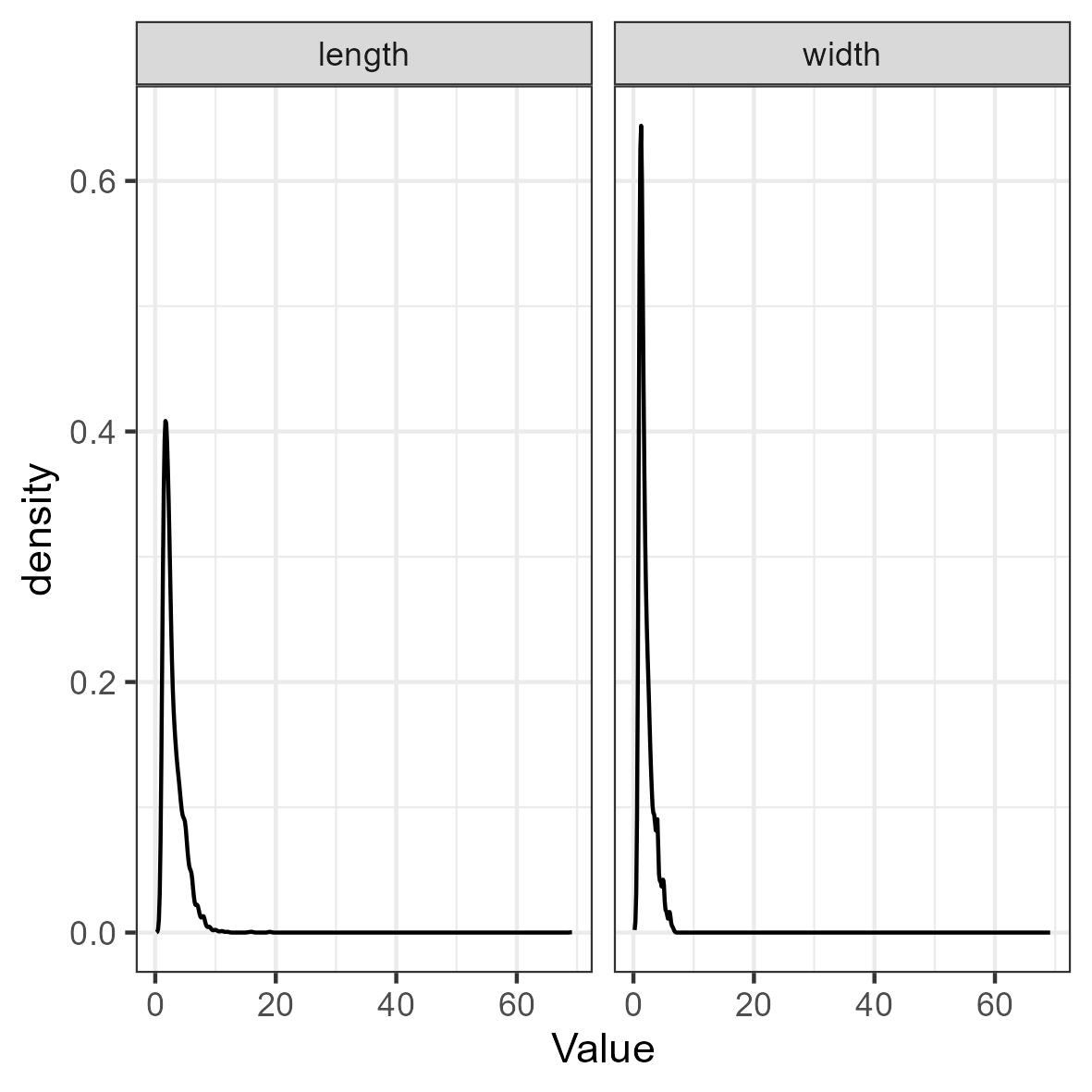Both the table above and the density plot indicate that the data are extremely right-skewed and kurtotic. With this in mind, it can be useful to transform and rescale the data. We will use a log transformation. df_plot$Value <- log(df_plot$Value) ggplot(df_plot, aes(x = Value)) + geom_density() + facet_wrap(~Variable) + theme_bw()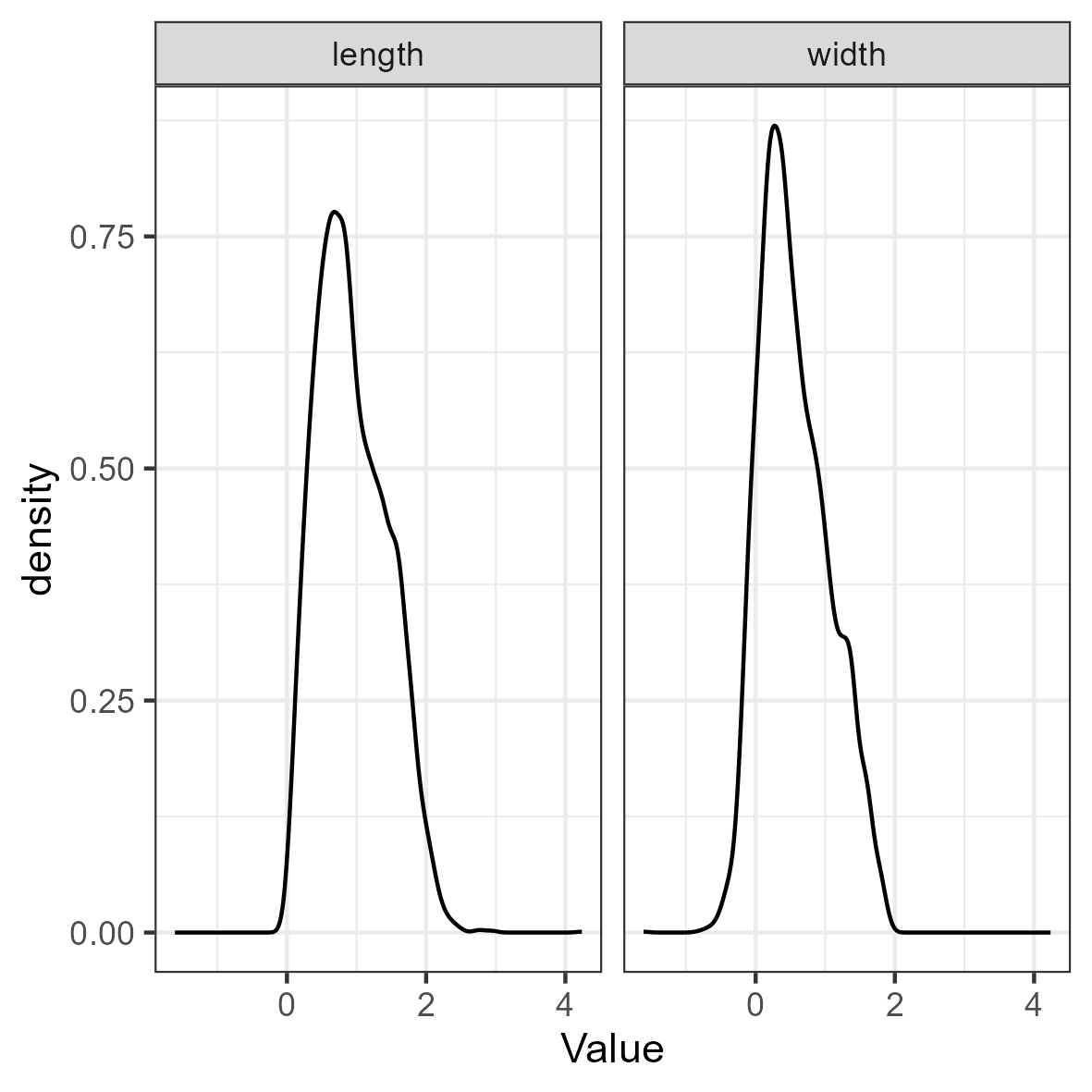The log transformation addresses the aforementioned concerns regarding skew and kurtosis. To confirm this, reshape the data to wide format and examine a scatterplot: df <- reshape(df_plot, direction = "wide", v.names = "Value")[, -1] names(df) <- gsub("Value.", "", names(df), fixed = TRUE) ggplot(df, aes(x = length, y = width)) + geom_point(alpha = 0.1) + theme_bw()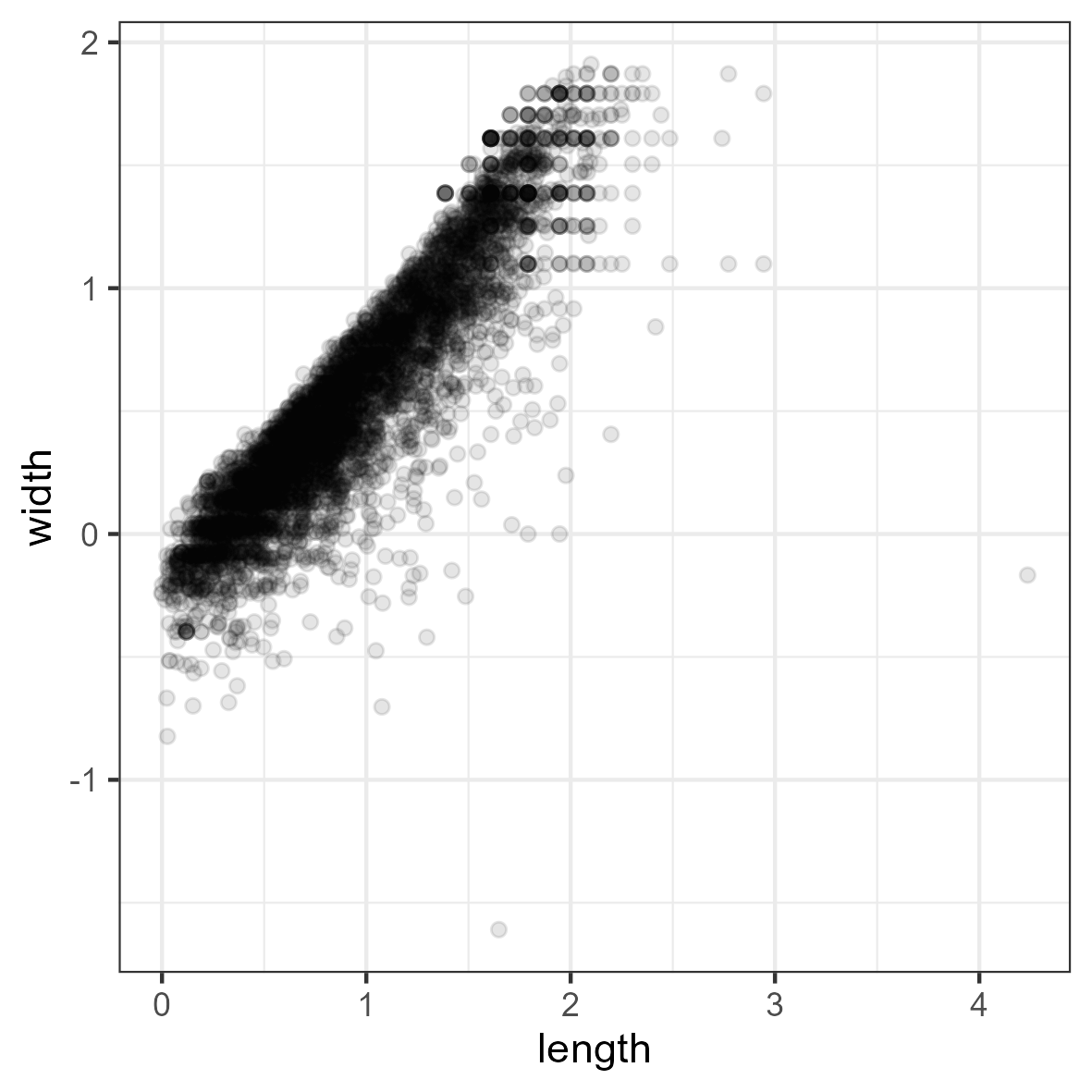## Conducting Latent Profile Analysis As all variables are continuous, we can use the convenience function tidySEM::mx_profiles(), which is a wrapper for the generic function mx_mixture() optimized for continuous indicators. Its default settings are appropriate for LPA, assuming fixed variances across classes and zero covariances. Its arguments are data and number of classes. All variables in data are included in the analysis, which is why we first selected the indicator variables. The models are estimated using simulated annealing, with start values determined via initial K-means clustering. As this is an exploratory LCA, we will conduct a rather extensive search across model specifications and number of classes. We will set the maximum number of classes $$K$$ to three to limit computational demands. We set a seed to ensure replicable results. As the analysis takes a long time to compute, it is prudent to save the results to disk immediately, so as not to lose them. For this, we use the function saveRDS(). We can later use res <- readRDS("res_gmm.RData") to load the analysis from the file. set.seed(123) res <- mx_profiles(data = df, classes = 1:3, variances = c("equal", "varying"), covariances = c("equal", "varying"), expand_grid = TRUE) saveRDS(res, "res_gmm.RData") ## Class Enumeration To compare the fit of the estimated models, we create a model fit table using table_fit(). We will use the BIC for class enumeration. fit <- table_fit(res) First, we determine whether any models can be disqualified. There were no indications of convergence problems during estimation, so this is not a reason to disqualify solutions. Next, we check for global and local identifiability. The global ratio of observations per parameter is large, as the minimum np_ratio is 244. The smallest ratio of class size to class-specific parameters is 18 (see np_local), which is no cause for concern. tbl <- fit[, c("Name", "LL", "Parameters", "BIC", "Entropy", "prob_min", "n_min", "np_ratio", "np_local")] names(tbl) <- c("Name", "LL", "p", "BIC", "Ent.", "p_min", "n_min", "np_ratio", "np_local") knitr::kable(tbl, caption = "Model fit table.") Model fit table. Name LL p BIC Ent. p_min n_min np_ratio np_local equal var, equal cov 1 -3389 5 6820 1.00 1.00 1.00 1121 1121 equal var, equal cov 2 -3082 8 6234 0.72 0.86 0.31 701 494 equal var, equal cov 3 -3030 11 6155 0.67 0.71 0.17 510 313 free var, equal cov 1 -3389 5 6820 1.00 1.00 1.00 1121 1121 free var, equal cov 2 -2545 10 5176 0.64 0.51 0.09 560 108 free var, equal cov 3 -2257 15 4643 0.68 0.54 0.06 374 83 equal var, free cov 1 -3389 5 6820 1.00 1.00 1.00 1121 1121 equal var, free cov 2 -2552 9 5181 0.65 0.52 0.09 623 121 equal var, free cov 3 -2368 13 4848 0.56 0.51 0.02 431 27 free var, free cov 1 -3389 5 6820 1.00 1.00 1.00 1121 1121 free var, free cov 2 -2575 11 5245 0.56 0.81 0.40 510 448 free var, free cov 3 -2111 17 4370 0.65 0.51 0.04 330 41 However, note that we have a very large sample, and for many models, the smallest class comprises only a very small percentage of the total sample. Since the purpose of this analysis is to better represent the distribution of ocean microplastics, we can wonder whether it makes sense to allow for classes that only describe a small percentage of the cases. We therefore only consider solutions that capture at least 10% of the sample. Another interesting characteristic of this data is that the BIC and the entropy are strongly correlated. The raw correlation between these two metrics is .66, cor(fit$BIC, fit$Entropy). If we omit the 1-class models, for which entropy is technically not defined, the correlation is even as high as .85, cor(fit$BIC[!fit$Classes == 1], fit$Entropy[!fit$Classes == 1]). This strong correlation indicates that an increase in fit comes with a decrease in class separability. This illustrates why entropy should not be treated as a model fit criterion. It also illustrates that criteria for class enumeration should be explicit, because we will likely come to a different decision depending on which criteria are used. As mentioned before, we drop models with < 10% of cases in the smallest class: fit <- fit[!fit$n_min < 0.1, ]

If our strategy is to optimize fit, we can examine the fit table above, or plot a scree plot for the BIC by calling plot(fit). Note that, due to the large sample size, all ICs give identical conclusions.

plot(fit) + theme(axis.text.x = element_text(angle = 90, vjust = 0.5,
hjust = 1))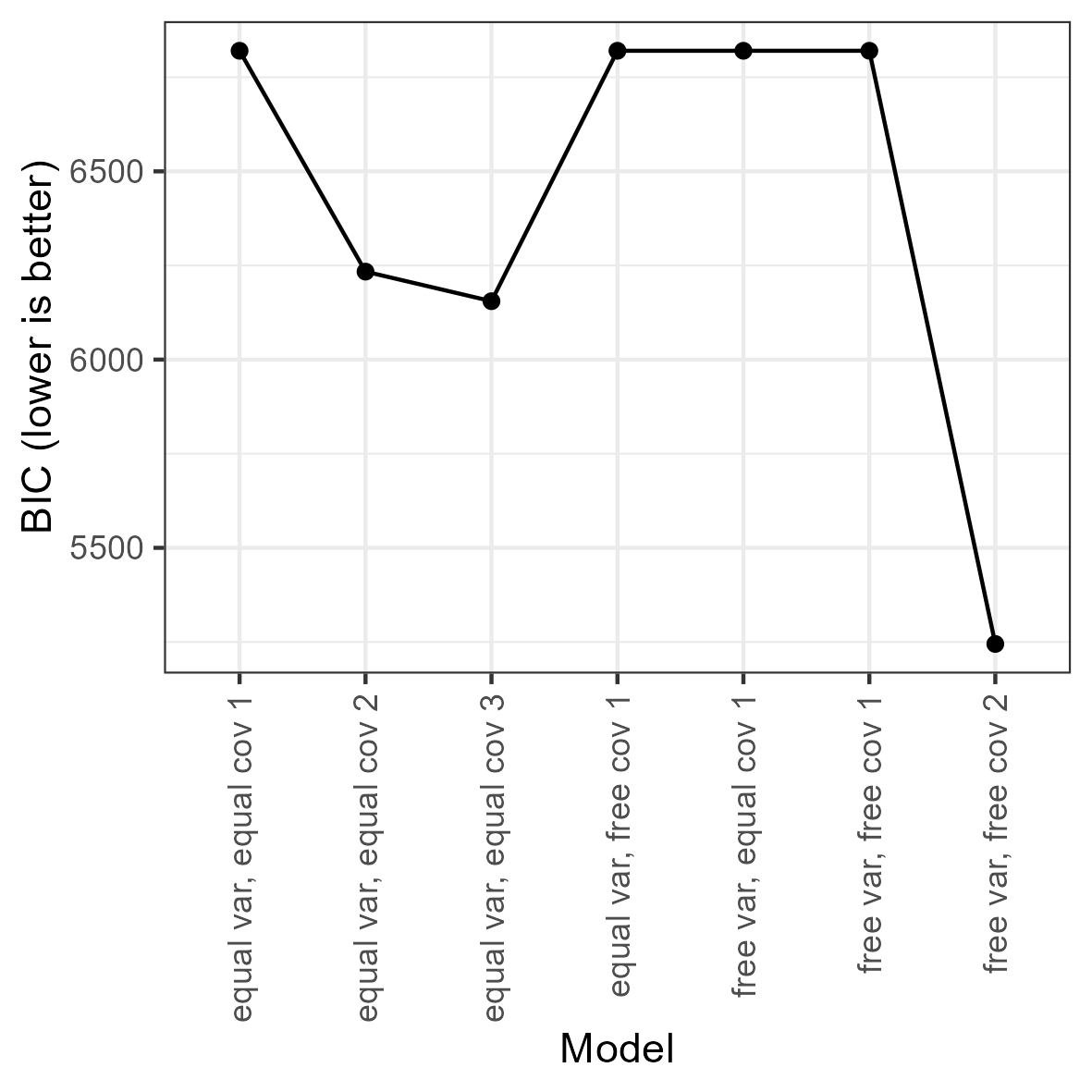Bivariate profile plot

Looking at the blocks of 1-4 class models for each model specification, it appears that the BIC keeps decreasing with the addition of more classes. Across the blocks, the BIC keeps decreasing with increasingly complex model specifications.

Out of the 16 models that remain after removing those with < 10% of cases in the smallest class, one model stands out: The 2-class model with free (co)variances. We thus select this as our final model.

## Interpreting the Final Class Solution

We here request the estimates (est) and standardized estimates std_est, because the latter allows us to interpret the correlations between length and width. Note that standard errors and p-values are relatively uninformative: With a sample size of 5606, every parameter is significantly different from zero.

res_bic <- res[["free var, free cov 2"]]
cp <- class_prob(res_bic)
results <- table_results(res_bic, columns = c("label", "est",
"std_est"))
results
Results of a 2-class model with free (co)variances
label est
Means.length 0.65
Means.width 0.34
Variances.length 0.10
Covariances.length.WITH.width 0.09
Variances.width 0.10
Means.length 1.33
Means.width 0.86
Variances.length 0.19
Covariances.length.WITH.width 0.20
Variances.width 0.28
mix2.weights[1,1] 1.00
mix2.weights[1,2] 0.75

Interpreting the results is facilitated by examining a plot of the model and data. Relevant plot functions are plot_bivariate(), plot_density(), and plot_profiles(). However, we omit the density plots, because plot_bivariate() also includes them.

plot_bivariate(res_bic)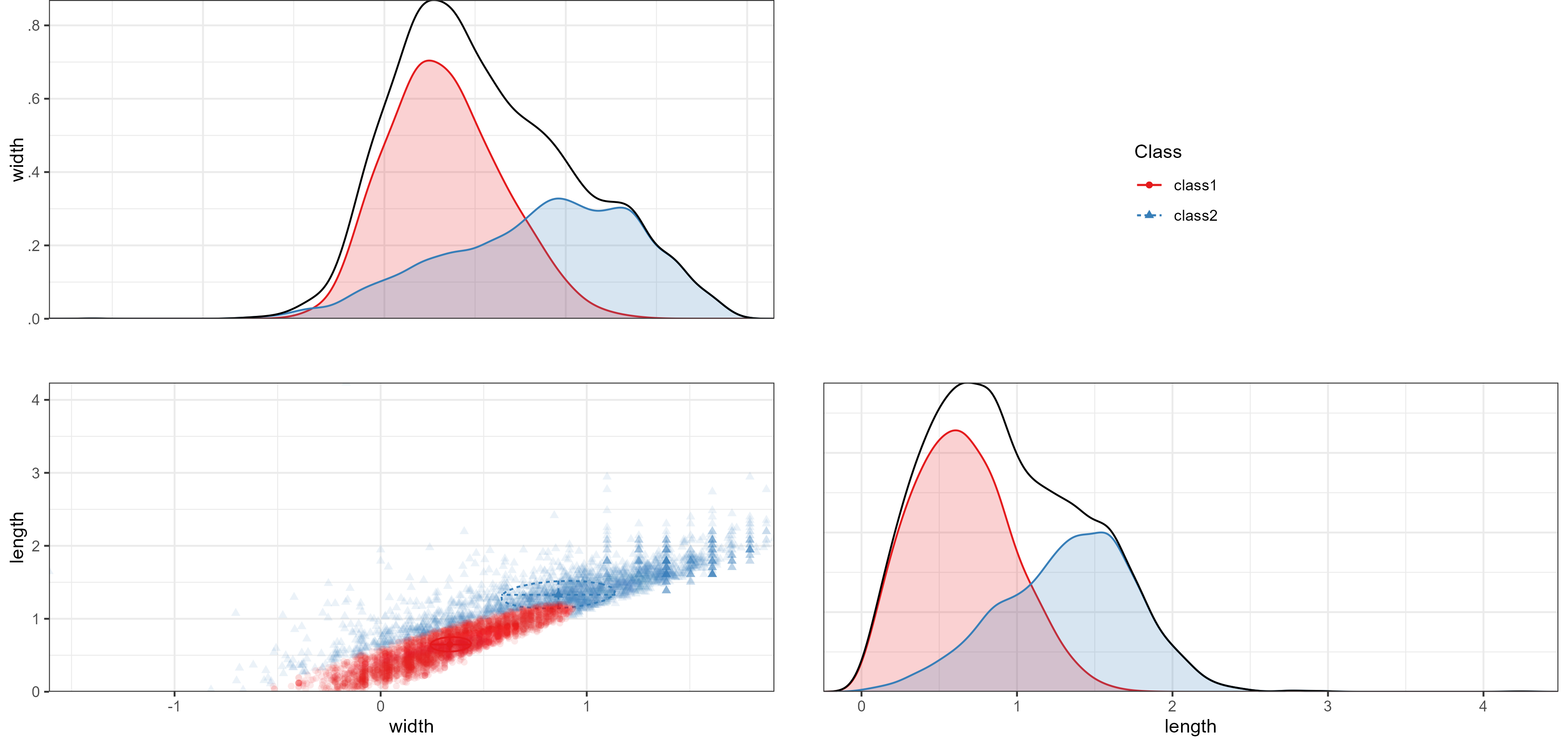Bivariate profile plot

On the diagonal of the bivariate plot are weighted density plots: normal approximations of the density function of observed data, weighed by class probability. On the off-diagonal are plots for each pair of indicators, with the class means indicated by a point, class standard deviations indicated by lines, and covariances indicated by circles.

The bivariate and marginal plots show that the classes are not clearly separable, as also evident from the low entropy. At the same time however, it is clear that the observed distributions are non-normal, and the second class accounts for some of this non-normality (there is a smaller ‘bump’ to the right of the mode, which could be the mean of a second normal distribution). The first class (57%) accounts for smaller fragments, and the second class (43%) accounts for some of the right-skew in fragments’ length and width. We label class 1 as small fragments, and class 2 as larger fragments.

It also appears that the correlation between length and width is stronger for small fragments than for large fragments. To test the difference, use wald_test(res_bic, hypothesis = "c11 = c21"). Results indicate that the correlation is indeed significantly larger for small fragments ($$r = .92$$) than for larger fragments ($$r = .85$$), $$\chi^2(1) = 11.56, p < .001$$. Thus, small fragments are more coextensive than large fragments.

There are, however, concerns about the interpretability of this solutions: the entropy is .56 and the minimum classification probability is.81. This is because of substantial overlap in the distributions of the two classes.

## Auxiliary Analyses

Finally, we may want to compare the different classes on auxiliary variables or models. The BCH() function applies three-step analysis, which compares the classes using a multi-group model, controlling for classification error. For example, we can test whether polymer type differs between the two classes. Because polymer type is a nominal variable, we must convert it to dummies and estimate a threshold for each dummy:

df_pt <- mx_dummies(df_analyze\$poly_type)
aux_pt <- BCH(res_bic, model = "poly_typeOther | t1
poly_typePE | t1
poly_typePP | t1",
data = df_pt)
aux_pt <- mxTryHardOrdinal(aux_pt)

To obtain an omnibus likelihood ratio test of the significance of the differences in polymer type across classes, use lr_test(aux_pt). The results indicate that there are significant differences in polymer types across classes, $$\Delta LL(3) = 17.14, p < .001$$. The results can be reported in probability scale using table_prob(aux_pt). To test differences for specific polymer types, we can use Wald tests:

wald_test(aux_pt, "class1.Thresholds[1,1] = class2.Thresholds[1,1];
class1.Thresholds[1,2] = class2.Thresholds[1,2];
class1.Thresholds[1,3] = class2.Thresholds[1,3]")

The results indicate that there is no significant difference in the prevalence of “Other” polymer types across classes. However, PE is significantly more prevalent in class 1, and PP is significantly more prevalent in class 2.